# pygmt.Figure.colorbar

Figure.colorbar(*, frame=None, cmap=None, position=None, box=None, truncate=None, shading=None, projection=None, equalsize=None, region=None, verbose=None, scale=None, zfile=None, panel=None, perspective=None, transparency=None, **kwargs)

Plot colorbars on figures.

Both horizontal and vertical colorbars are supported. For CPTs with gradational colors (i.e., the lower and upper boundary of an interval have different colors) we will interpolate to give a continuous scale. Variations in intensity due to shading/illumination may be displayed by setting the `shading` parameter. Colors may be spaced according to a linear scale, all be equal size, or by providing a file with individual tile widths.

Full option list at https://docs.generic-mapping-tools.org/latest/colorbar.html

Aliases:

• B = frame

• C = cmap

• D = position

• F = box

• G = truncate

• J = projection

• L = equalsize

• R = region

• V = verbose

• W = scale

• Z = zfile

• c = panel

• p = perspective

• t = transparency

Parameters
• frame (str or list) – Set colorbar boundary frame, labels, and axes attributes.

• cmap (str) – File name of a CPT file or a series of comma-separated colors (e.g., color1,color2,color3) to build a linear continuous CPT from those colors automatically.

• position (str) – [g|j|J|n|x]refpoint[+wlength[/width]][+e[b|f][length]][+h|v][+jjustify][+m[a|c|l|u]][+n[txt]][+odx[/dy]]. Define the reference point on the map for the color scale using one of four coordinate systems: (1) Use g for map (user) coordinates, (2) use j or J for setting refpoint via a 2-character justification code that refers to the (invisible) map domain rectangle, (3) use n for normalized (0-1) coordinates, or (4) use x for plot coordinates (inches, cm, etc.). All but x requires both `region` and `projection` to be specified. Append +w followed by the length and width of the colorbar. If width is not specified then it is set to 4% of the given length. Give a negative length to reverse the scale bar. Append +h to get a horizontal scale [Default is vertical (+v)]. By default, the anchor point on the scale is assumed to be the bottom left corner (BL), but this can be changed by appending +j followed by a 2-character justification code justify.

• box (bool or str) – [+cclearances][+gfill][+i[[gap/]pen]][+p[pen]][+r[radius]][+s[[dx/dy/][shade]]]. If set to `True`, draw a rectangular border around the color scale. Alternatively, specify a different pen with +ppen. Add +gfill to fill the scale panel [Default is no fill]. Append +cclearance where clearance is either gap, xgap/ygap, or lgap/rgap/bgap/tgap where these items are uniform, separate in x- and y-direction, or individual side spacings between scale and border. Append +i to draw a secondary, inner border as well. We use a uniform gap between borders of 2p and the MAP_DEFAULTS_PEN unless other values are specified. Append +r to draw rounded rectangular borders instead, with a 6p corner radius. You can override this radius by appending another value. Finally, append +s to draw an offset background shaded region. Here, dx/dy indicates the shift relative to the foreground frame [4p/-4p] and shade sets the fill style to use for shading [Default is `"gray50"`].

• truncate (list or str) – zlo/zhi. Truncate the incoming CPT so that the lowest and highest z-levels are to zlo and zhi. If one of these equal NaN then we leave that end of the CPT alone. The truncation takes place before the plotting.

• scale (float) – Multiply all z-values in the CPT by the provided scale. By default, the CPT is used as is.

• shading (str or list or bool) – Add illumination effects. Passing a single numerical value sets the range of intensities from -value to +value. If not specified, 1 is used. Alternatively, set `shading=[low, high]` to specify an asymmetric intensity range from low to high. [Default is no illumination].

• equalsize (int or float or str) – [i][gap]. Equal-sized color rectangles. By default, the rectangles are scaled according to the z-range in the CPT (see also `zfile`). If gap is appended and the CPT is discrete each annotation is centered on each rectangle, using the lower boundary z-value for the annotation. If i is prepended the interval range is annotated instead. If `shading` is used each rectangle will have its constant color modified by the specified intensity.

• zfile (str) – File with colorbar-width per color entry. By default, the width of the entry is scaled to the color range, i.e., z = 0-100 gives twice the width as z = 100-150 (see also `equalsize`). Note: The widths may be in plot distance units or given as relative fractions and will be automatically scaled so that the sum of the widths equals the requested colorbar length.

• verbose (bool or str) –

Select verbosity level [Default is w], which modulates the messages written to stderr. Choose among 7 levels of verbosity:

• q - Quiet, not even fatal error messages are produced

• e - Error messages only

• w - Warnings [Default]

• t - Timings (report runtimes for time-intensive algorithms)

• i - Informational messages (same as `verbose=True`)

• c - Compatibility warnings

• d - Debugging messages

• panel (bool or int or list) – [row,col|index]. Select a specific subplot panel. Only allowed when in subplot mode. Use `panel=True` to advance to the next panel in the selected order. Instead of row,col you may also give a scalar value index which depends on the order you set via `autolabel` when the subplot was defined. Note: row, col, and index all start at 0.

• perspective (list or str) – [x|y|z]azim[/elev[/zlevel]][+wlon0/lat0[/z0]][+vx0/y0]. Select perspective view and set the azimuth and elevation angle of the viewpoint [Default is `[180, 90]`]. Full documentation is at https://docs.generic-mapping-tools.org/latest/gmt.html#perspective-full.

• transparency (int or float) – Set transparency level, in [0-100] percent range [Default is `0`, i.e., opaque]. Only visible when PDF or raster format output is selected. Only the PNG format selection adds a transparency layer in the image (for further processing).

Example

```>>> import pygmt
>>> # Create a new figure instance with pygmt.Figure()
>>> fig = pygmt.Figure()
>>> # Create a basemap
>>> fig.basemap(region=[0, 10, 0, 3], projection="X10c/3c", frame=True)
>>> # Call the colorbar method for the plot
>>> fig.colorbar(
...     # Set cmap to the "roma" CPT
...     cmap="roma",
...     # Label the x-axis "Velocity" and the y-axis "m/s"
...     frame=["x+lVelocity", "y+lm/s"],
... )
>>> # Show the plot
>>> fig.show()
```

## Examples using `pygmt.Figure.colorbar`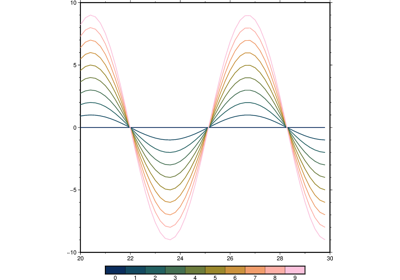Line colors with a custom CPT

Line colors with a custom CPT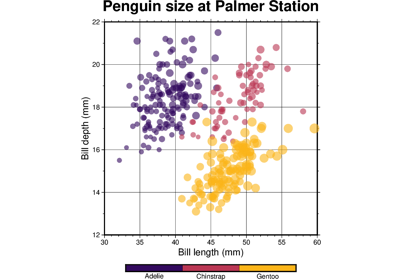Color points by categories

Color points by categories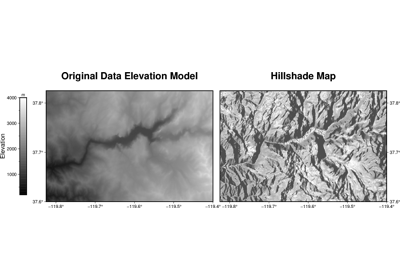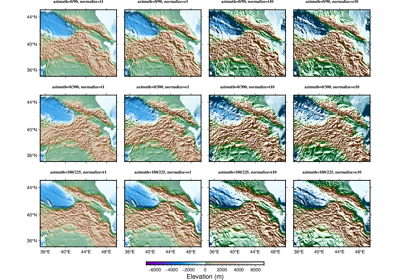Calculating grid gradient with custom azimuth and normalize parameters

Calculating grid gradient with custom azimuth and normalize parameters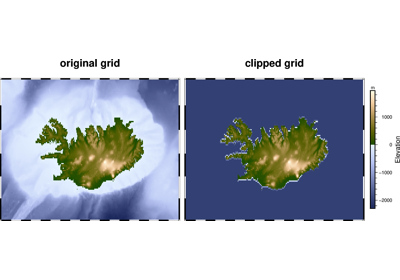Clipping grid values

Clipping grid values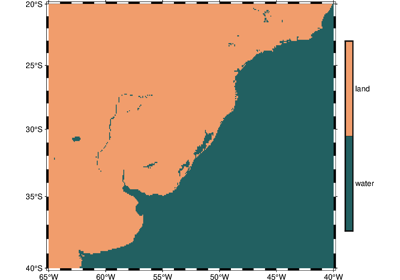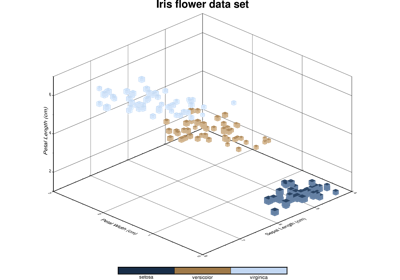3-D Scatter plots

3-D Scatter plots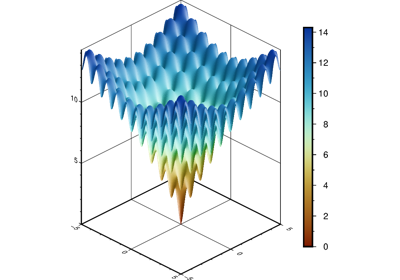Plotting a surface

Plotting a surface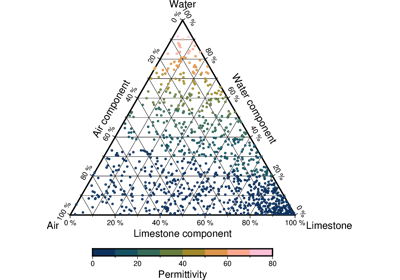Ternary diagram

Ternary diagram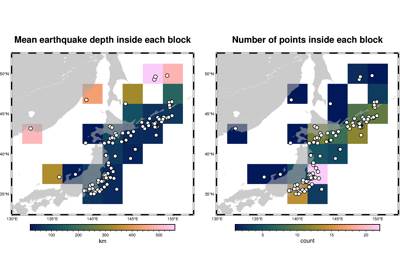Blockmean

Blockmean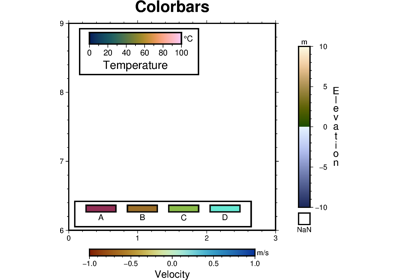Colorbar

Colorbar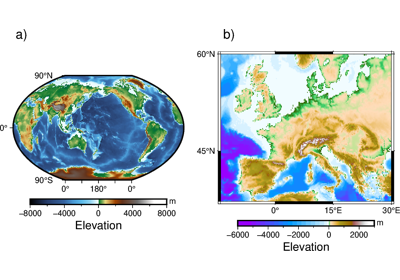Multiple colormaps

Multiple colormaps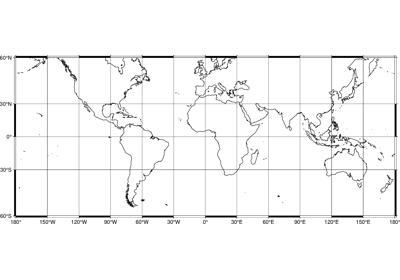Frames, ticks, titles, and labels

Frames, ticks, titles, and labels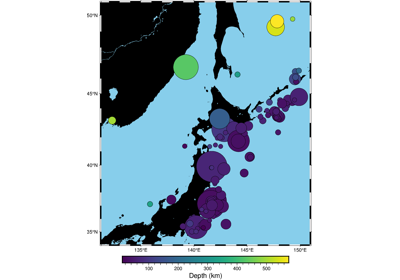Plotting data points

Plotting data points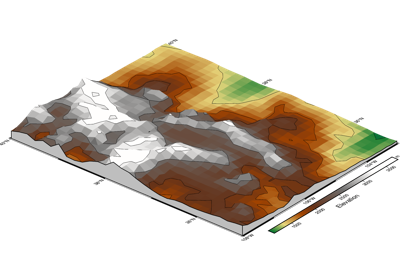Creating a 3-D perspective image

Creating a 3-D perspective image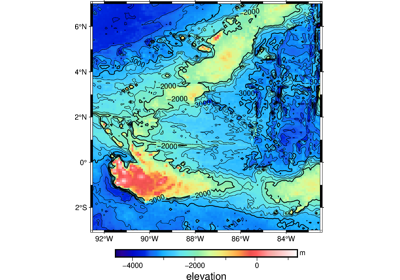Creating a map with contour lines

Creating a map with contour lines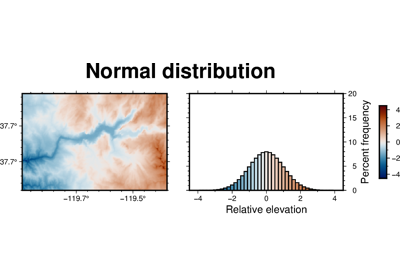Performing grid histogram equalization

Performing grid histogram equalization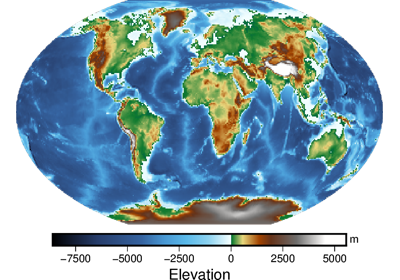Plotting Earth relief

Plotting Earth relief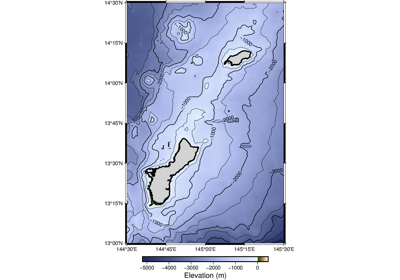2. Create a contour map

2. Create a contour map